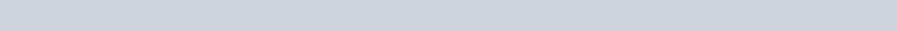Inorganic Chemistry Questions

CHEM 441

GMU Honor Code strictly enforced. You may not discuss this assignment with students in your class, former students, any faculty members whatsoever, staff, etc. Students that engage in honor code violations will be reported to the Office of Academic Integrity, a grade of “zero” recorded for the assignment, along with recommendation of “suspension from GMU”.

1. While d-electrons in many transition metal compounds may not be involved directly in bond formation, they nevertheless exert a considerable influence on structure. Explain.

1. The structure of the so-called 2-1-4 superconductor, La2-xSrxCuO4, is very similar to that shown for

Sr2TiO4, except that the axial Cu-O distances perpendicular to the sheet are quite long (~2.45Å). Rationalize the observation that as x increases the copper-oxygen distance in the CuO2 plane decreases.

(1.9035(1)Å for x=0, 1.8896(1)Å for x=0.15.)Idealized crystal structures of a perovskite (e.g., SrTiO3: Sr—open circles; TiO6octahedra), b layered perovskitetype (e.g., Sr2TiO4: Sr—open circles) and La2-xSrxCuO4 open network and looking down z-axis.

1. The frequency of the symmetrical M-O stretching vibration of the octahedral aqua ions [M(OH2)6]2+ increases along the series Ca2+<Mn2+<Ni2+. How does this trend relate to acidity?

1. Identify the lattice planes shown below.1. What is the probable lattice type of crystalline substances that give the following observed reflections? Body centered? Face centered? Etc.?

 (a) 110, 200,103, 202, 211 (b) 111, 200, 113, 220, 222 (c) 100, 110, 111, 200, 210 (d) 001, 110, 200, 111, 201

1. The physical properties of transition metal oxide compounds continue to intrigue scientist and engineers. Two compounds of interest are TiO2 and RuO2. The table below shows features of each transition metal.

 Property Titanium Ruthenium Electron configuration of element [Ar]4s23d2 [Kr]5s24d6 Oxidation state of ion 4+ 4+ Ionic radius 0.605 Å 0.62 Å

Provide a plausible explanation for why TiO2 is a wide band gap semiconductor, but RuO2 is a metallic conductor. The two materials have the same crystal structure (rutile structure).

1. White tin, the stable form at room temperature, has a tetragonal unit cell with a = 5.8197 Å and c = 3.1749 Å. When cooled below room temperature, the white tin converts to gray tin, which has the cubic diamond structure. Gray tin has Z = 8 atoms/unit cell, whereas the metallic form of white tin has Z = 4 atoms/unit cell. The atomic weight of tin is 116.69 g/mol. Based on the preceding information, calculate the density of gray tin and white tin. Show all work. Which structure would you expect to exhibit a higher degree of conductivity and why? What does this imply about the band gap of the solid compound?1. The two polymorph modifications of LiFe5O8, fine particles were successfully prepared by ceramic technique at pre-sintering temperature of 500°C and the pre-sintered material was crushed and sintered finally in the open air at 1000°C. The structural and microstructural evolutions of the nanophase were studied using powder X-ray diffraction (PXRD). The two polymorphous LiFe5O8 includes the ordered phase, termed α-LiFe5O8, with a primitive cubic unit cell (space group P4332, a=8.337 Å) in which the Fe3+ ions are at octahedral 12d and tetrahedral 8c sites, and Li+ ions occupy only the octahedral 4b positions in the cubic primitive unit cell and disordered face centered cubic structure, termed β-LiFe5O8 (space group Fd-3m, a = 8.3333 Å), The refinement of XRD results revealed the presence of mixed and nearly inverse spinel nanocrystalline Li-ferrite phases. Determine the hkl values for the peaks indicated below (ovals) for the β-LiFe5O8 phase. l = 1.5405 Å.2q (degrees) q (degrees) d-spacing (Å) h2 + k2 + l2 (h k l)

SHOW ALL CALCULATION STEPS INVOLVED TO DETERMINE THE VALUES for the peak at 2q = ~35.5°

***********************************************************************

Bragg’s Law: λ = 2dhkl sin θhkl

The interplanar spacing, d, for a given hkl reflection is given by the unit cell dimensions:

12 ” h2 + k22 + l2% ; cubic 12 =”\$ h2 +2k2%’ +\$” l22%’ ; tetragonal

=\$ ‘

d # a & d # a & # c &

12 =”\$ h22%’ +”\$ k22‘% +”\$ l22%’ ; orthorhombic 12 =\$” 4%’\$” h2 + hk2 + k2%’ +\$” l22%’ ; hexagonal

d # a & # b & # c & d # 3&# a & # c &

12 =#% 12 &(#% h22 + k2sin2 2β+ l22 − 2hlcosβ&( ; monoclinic

d \$ sin β’\$ a b c ac

πr π( λ

3 43 2 )3 = 33.5 3 = 33.5(Vdirect3 )

4

3

N = =

V Vrecip Vrecip *λ λ

Vrecip = volume of the reciprocal cell

Vdirect = volume of direct cell

1 2sinθ| S |= = where |S| is the scattering factor dhkl λ r =

𝑀𝑍

𝜌=

𝑁&𝑉

Where M=molecular weight (g/mol); Z=number of formula units per unit cell; NA = Avogadro’s number; rho (r ) = density (g/cm3); V = volume (cm3)